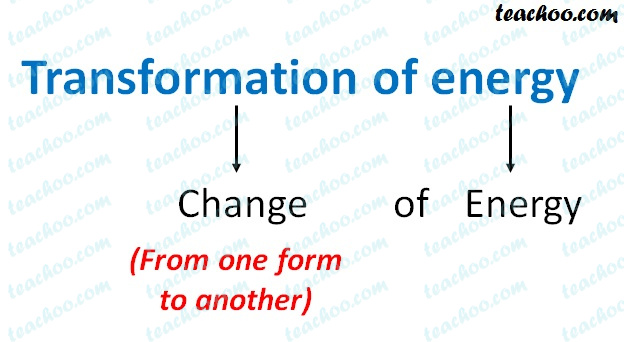Concepts

Class 9
Chapter 11 Class 9 - Work and Energy (Term 2)

## What is Transformation of Energy

It is change of energy from one form to another## Examples of Transformation of Energy

### Dropping of brick from HeightIn this case, potential energy gets transformed into kinetic enrrgy

Potential Energy → Kinetic Energy

Explanation

Suppose brick is lying at the roof of House

It currently has potential energy

When it is dropped from roof to earth

Its potential energy gets converted into kinetic energy

As it falls down on earth, its speed increases (this is because its potential energy keeps on decreasing and kinetic energy keeps

on increasing)

Thus there is transformation of Potential energy into kinetic energy when

## Brick Thrown upwardsIn this case, kinetic energy is transformed into potential energy

Kinetic Energy → Potential Energy

Explanation

When a brick is thrown upwards,its speed keeps on decreasing

This is because kinetic energy in brick keeps in decreasing and potential energy keeps on increasing

Thus there is transformation of Kinetic energy into potential energy when a brick is thrown upwards.

## Hydroelectric Power HouseIn this case, potential energy of water is transformed into kinetic energy which later gets transformed into Electrical Energy

Potential Energy → Kinetic Energy → Electric Energy

Explanation

in a hydroelectric power house,electricity is generated with the help of water

Water is made to fall on turbines at a great speed which make turbines move (generating kinetic energy)

This kinetic energy is convered into electrical energy with the help of generator

## Energy Changes When a Body Does Work

When we do some work, we feel tired

This is because we lose energy

This energy which we lose is gained by some other body

### Example 1

When we push a heavy Box, we feel tired and lose energy

This energy which we lose is gained by box in the form of kinetic energy
This kinetic energy makes the box move

### Example 2

When we rub our hands,it produces heat

When we rub our hands, the kinetic energy is produced while rubbing the hands.

This kinetic energy is produced by lose of some energy from our body.

Later, the kinetic energy of our hands converted into heat energy.

### Example 3

When we lift a heavy box

When we lift a box, we feel tired and lose energy.

This energy which we lose is gained by the box in the form of potential energy.

## Questions

NCERT Question 21 - A freely falling object eventually stops on reaching the ground. What happened to its kinetic energy?